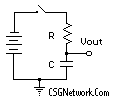# Resistance, Capacitance, Voltage And Time Calculator

This calculator requires the use of Javascript enabled and capable browsers. This calculator is designed to calculate any one value in the group of Voltage, Capacitance, Resistance, Time of charge, and Instant Voltage. In the schematic rendering, the time required for the capacitor to charge to 63.2% of the battery voltage after the switch is closed is the product of the resistance and capacitance T=(R*C). For example, a rather common circuit is in which a 100 uF capacitor and a 100K resistor would require 10 seconds to charge to 7.6 volts using a 12 volt battery. Our calculator can be used to determine the capacitor voltage at other times after the switch in the circuit is closed (turned on). Any of the five variables can be determined by supplying the other four. For example, in the calculator defaults, a 1 Farad capacitor charging through a 1 ohm resistor and 1 volt battery will reach about 777 millivolts in 1.5 seconds. From left to right, the entries would be 1, .001, 1000000, 1500 with the Instantaneous Voltage field left blank, or zero. The result should be about 0.777. Only one field should be blank or a 0 value before each calculation. The general formula for these types of calculations is:

Vc = V * (1- e^(-t / R*C))

Where Vc is the capacitor voltage, V is the supply voltage from battery or other source, R is the resistance, C is the Capacitance, t is the time, and e = 2.71828 which is a constant in our calculator.Designation Value Required Data - Any Four Values Supply Voltage Volts Resistance KOhms Capacitance Microfarads Time Milliseconds Instantaneous Voltage Volts
Version 1.4.3

 Leave us a question or comment on FacebookSearch or Browse Our Site